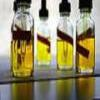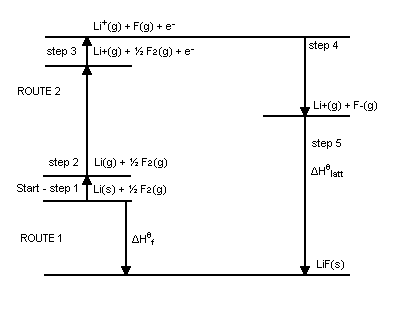#### You may also like### Mathematical Issues for Chemists

A brief outline of the mathematical issues faced by chemistry students.### Reaction Rates

Explore the possibilities for reaction rates versus concentrations with this non-linear differential equation### Catalyse That!

Can you work out how to produce the right amount of chemical in a temperature-dependent reaction?

# What Salt?

##### Age 16 to 18Challenge Level

Have you ever wondered why common salt is NaCl rather than NaCl$_2$ or NaCl$_3$? Here's your chance to investigate this mystery!

In case you haven't seen a Born-Haber cycle before, one is illustrated below for LiF:[http://en.wikipedia.org/wiki/File:BornHaberLiF.PNG]

Using the data below, construct a Born-Haber cycle for NaCl and NaCl$_2$ . Calculate the lattice enthalpy for NaCl, and the enthalpy of formation of NaCl$_2$

$\Delta H_f(NaCl)$ = -411 kJ mol$^{-1}$
$\Delta H_{latt}(NaCl_2)$ =-3360 kJ mol$^{-1}$
$IE_1(Na)$ = +496 kJ mol$^{-1}$
$IE_2(Na)$ = +4563 kJ mol$^{-1}$
$EA_1(Cl)$ = -349 kJ mol$^{-1}$
$\Delta H_{sub}(Na_{(s)})$ = +108 kJ mol$^{-1}$
$\Delta H_{at}(\frac{1}{2}Cl_{2\ (g)})$ = +121 kJ mol$^{-1}$

From looking at the signs and relative magnitudes of the two calculated enthalpies of formation, can you explain why NaCl$_2$ is not formed?

What are the major contributing factors in the mathematical expression for the enthalpies of formation which makes the formation of NaCl$_2$ so unfavourable? What does this tell you about the likely formation of NaCl$_n$, where n > 2 ?

As you may have guessed by now, the contsruction of Born-Haber cycles are quite laborious, and also rely solely upon experimentally determined enthalpy values. In many cases these enthalpy values may not be present, and so a reasonable prediction of a lattice enthalpy can be calculated by the Born-Lande expression (which is shown below). This equation assumes that ions are hard spheres held together solely by electrostatic attractions.

$$E = -\frac{N_AMz^+z^-e^2}{4\pi \epsilon_0r_0}(1-\frac{1}{n})$$

where:
$E$ is the lattice enthalpy
$N_A$ is Avogadro's number ($6.023 \times 10^{23}$)
$z^+$ is the charge on the cation in electron units
$z^-$ is the charge on the anion in electron units
$e$ is the charge on an electron ($1.602 \times 10^{-19} C$)
$4\pi \epsilon_0 = 1.11 \times 10^{-10} C^2/(J m)$
$r_0$ is distance to closest ion
$n$ and $M$ are constants for a given lattice

Using the data below, use the Born-Lande equation to calculate the lattice enthalpies of AgCl, AgBr and AgI.

$M$ = -1.74756
$n_{AgCl}$ =10.5
$n_{AgBr}$ = 11
$n_{AgBr}$ = 12
$r_{Ag^+}$ =115 pm
$r_{Cl^-}$ = 181 pm
$r_{Br^-}$ = 196 pm
$r_{I^-}$ = 220 pm

$\Delta H_{latt}(AgCl)$ = -915 kJ mol$^{-1}$
$\Delta H_{latt} (AgBr)$ = -904 kJ mol$^{-1}$
$\Delta H_{latt} (AgI)$ = - 889 kJ mol$^{-1}$

Comparing these values of the lattice energies to those calculated using the Born-Haber cycle, for which of the lattices does the Born-Lande equation give a good approximation?

Why is there a degree of discrepancy between the two approaches for calculating the lattice enthalpy? Why does this discrepancy increase with larger halides?